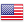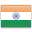Grade "A+" Accredited by NAAC with a CGPA of 3.46
Grade "A+" Accredited by NAAC with a CGPA of 3.46

# Machine Learning

Course ID
BHCS 18A
Level
Program
B.Sc. CS (Hons.)
Semester
Sixth
Credits
6.0
Paper Type
DSE - 4
Method
Lecture & Practical

## Unique Paper Code: Update Awaited

The course aims at introducing the basic concepts and techniques of machine learning so that a student can apply machine learning techniques to a problem at hand..

## Learning Outcomes:

At the end of the course, students should be able to:

• Differentiate between supervised and unsupervised learning tasks
• Differentiate between linear and non-linear classifiers.
• Describe theoretical basis of SVM.
• Implement various machine learning algorithms learnt in the course.

## Course Contents

Unit 1
Unit 2
Unit 3
Unit 4

Unit 1

Introduction: Basic definitions, Hypothesis space and inductive bias, Bayes optimal classifier and Bayes error, Occam’s razor, Curse of dimensionality, dimensionality reduction, feature scaling, feature selection methods.

Unit 2

Regression: Linear regression with one variable, linear regression with multiple variables, gradient descent, logistic regression, over-fitting, regularization. performance evaluation metrics, validation methods.

Unit 3

Classification: Decision trees, Naive Bayes classifier, k-nearest neighbor classifier, perceptron, multilayer perceptron, neural networks, back-propagation algorithm, Support Vector Machine (SVM), Kernel functions.

Unit 4

Clustering: Approaches for clustering, distance metrics, K-means clustering, expectation maximization, hierarchical clustering, performance evaluation metrics, validation methods.

### Practicals

#### Lab List 1

For practical Labs for Machine Learning, students may use softwares like MABLAB/Octave or Python. For later exercises, students can create/use their own datasets or utilize datasets from online repositories like UCI Machine Learning Repository (http://archive.ics.uci.edu/ml/).

1. Perform elementary mathematical operations in Octave/MATLAB like addition, multiplication, division and exponentiation

2. Perform elementary logical operations in Octave/MATLAB (like OR, AND, Checking for Equality, NOT, XOR).
3. Create, initialize and display simple variables and simple strings and use simple formatting for variable.
4. Create/Define single dimension / multi-dimension arrays, and arrays with specific values like array of all ones, all zeros, array with random values within a range, or a diagonal matrix.
5. Use command to compute the size of a matrix, size/length of a particular row/column, load data from a text file, store matrix data to a text file, finding out variables and their features in the current scope.
6. Perform basic operations on matrices (like addition, subtraction, multiplication) and display specific rows or columns of the matrix.
7. Perform other matrix operations like converting matrix data to absolute values, taking the negative of matrix values, additing/removing rows/columns from a matrix, finding the maximum or minimum values in a matrix or in a row/column, and finding the sum of some/all elements in a matrix.
8. Create various type of plots/charts like histograms, plot based on sine/cosine function based on data from a matrix. Further label different axes in a plot and data in a plot.
9. Generate different subplots from a given plot and color plot data.
10. Use conditional statements and different type of loops based on simple example/s.

#### Lab List 2

1. Perform vectorized implementation of simple matrix operation like finding the transpose of a matrix, adding, subtracting or multiplying two matrices.
2. Implement Linear Regression problem. For example, based on a dataset comprising of existing set of prices and area/size of the houses, predict the estimated price of a given house.
3. Based on multiple features/variables perform Linear Regression. For example, based on a number of additional features like number of bedrooms, servant room, number of balconies, number of houses of years a house has been built – predict the price of a house.
4. Implement a classification/ logistic regression problem. For example based on different features of students data, classify, whether a student is suitable for a particular activity. Based on the available dataset, a student can also implement another classification problem like checking whether an email is spam or not.
5. Use some function for regularization of dataset based on problem 14.
6. Use some function for neural networks, like Stochastic Gradient Descent or backpropagation- algorithm to predict the value of a variable based on the dataset of problem 14.

#### Text Books

Flach, P. (2015). Machine Learning: The Art and Science of Algorithms that Make Sense of Data. Cambridge University Press.
Mitchell, T.M. (2017). Machine Learning. McGraw Hill Education.

Christopher & Bishop, M. (2016). Pattern Recognition and Machine Learning. New York: Springer-Verlag
Haykins, S.O. (2010). Neural Networks and Learning Machines. 3rd edition. PHI.

#### Teaching Learning Process

Use of ICT tools in conjunction with traditional class room teaching methods
Interactive sessions
Class discussions

#### Assessment Methods

Written tests, assignments, quizzes, presentations as announced by the instructor in the class

#### Keywords

Machine learning, unsupervised learning, supervised learning, support vector machines, neural networks, classification, clustering.

Disclaimer: Details on this page are subject to change as per University of Delhi guidelines. For latest update in this regard please refer to the University of Delhi website here.Englishहिन्दी